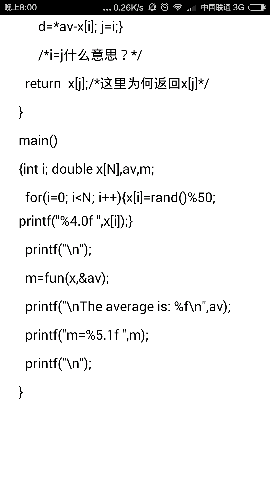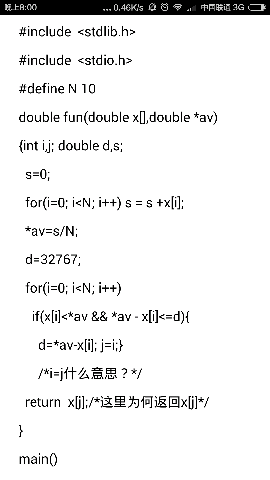/*用rand函数生成随机数，调用fun解决，生成平均值，和一个与平均值最接近的的小于平均数的值。*/1个回答

double fun（double x[],double *av）这个函数做了两步操作：

``````for(i=0;i<N;i++) s=s+x[i];
*av=s/N;

``````

`````` for(i=0;i<N;i++) // 遍历数组
if(x[i]<*av&&*av-x[i]<=d) //找出数组中小于平均值  且 与平均值差值最小的数 ，，其中d是差值
｛
d=*av-x[i];    // 记录与平均值最接近的一个数 与平均值的差值
j=i;       // 记录与平均值最接近的一个数在数组中的位置，，用变量j来记录
}
``````